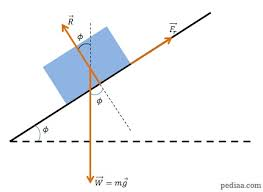## How to Calculate and Solve for Friction Angle | Rock MechanicsThe image above represents friction angle.

To compute for friction angle, two essential parameters are needed and these parameters are Uniaxial Compressive Strength (σc) and cohesion (c).

The formula for calculating the friction angle:

φ = 2tan-1(σc – 2c / σc + 2c)

Where;

φ = Friction Angle
σc = Uniaxial Compressive Strength
c = Cohesion

Let’s solve an example;
Find the friction angle when the uniaxial compressive strength is 11 and the cohesion is 22.

This implies that;

σc = Uniaxial Compressive Strength = 11
c = Cohesion = 22

φ = 2tan-1(σc – 2c / σc + 2c)
φ = 2tan-1(11 – 2(22) / 11 + 2(22))
φ = 2tan-1(11 – 44 / 11 + 44)
φ = 2tan-1(-33 / 55)
φ = 2tan-1(-0.6)
φ = 2(-30.96°)
φ = -61.927

Therefore,  the friction angle is -61.927.